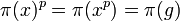Every normal subgroup satisfies the quotient-to-subgroup powering-invariance implication

This article gives the statement and possibly, proof, of an implication relation between two subgroup properties. That is, it states that every subgroup satisfying the first subgroup property (i.e., quotient-powering-invariant subgroup) must also satisfy the second subgroup property (i.e., powering-invariant subgroup)
View all subgroup property implications | View all subgroup property non-implications
Get more facts about quotient-powering-invariant subgroup|Get more facts about powering-invariant subgroup

Statement

Original formulation

Suppose$G$ is a group and$H$ is a normal subgroup of$G$. Suppose$p$ is a prime number such that$G$ is powered over$p$ (every element of$G$ has a unique$p^{th}$ root). Suppose that the quotient group$G/H$ is also powered over$p$. Then,$H$ is also powered over$p$.

Corollary formulation

Suppose$G$ is a group and$H$ is a normal subgroup of$G$ that is a quotient-powering-invariant subgroup: if$G$ is powered over a prime$p$ (i.e., every element of$G$ has a unique$p^{th}$ root), so is the quotient group$G/H$.

Then,$H$ is also a powering-invariant subgroup of$H$: if$G$ is powered over a prime$p$, so is$H$.

Proof

Proof idea

The idea is to apply the uniqueness of$p^{th}$ roots in the quotient group to the case of the$p^{th}$ roots of the identity element of the quotient group.

Proof details for original formulation

Given: A group$G$, a normal subgroup$H$ of$G$. A prime$p$ such that both$G$ and$G/H$ are powered over$p$. An element$g \in H$.

To prove: There is a unique element$x \in H$ such that$x^p = g$.

Proof: Let$\pi:G \to G/H$ be the quotient map.

Step no. Assertion/construction Facts used Given data used Previous steps used Explanation
1 There exists a unique element$x \in G$ such that$x^p = g$.$G$ is powered over$p$,$x \in H \le G$. Direct.
2 The$x$ of Step (1) is in$H$.$G/H$ is powered over$p$,$g \in H$. Step (1) Since$G/H$ is powered over$p$, there is a unique element whose$p^{th}$ power is the identity element of$G/H$. This unique element must therefore be the identity element of$G/H$. But we also have that$\pi(x)^p = \pi(x^p) = \pi(g)$ is the identity element of$G/H$ since$g \in H$. This forces that$\pi(x)$ is the identity element of$G/H$, so$x \in H$.

Proof for corollary formulation

This is direct from the original formulation once we note that:$G$ is powered over$p$ and$H$ is quotient-powering-invariant in$G$$\implies$$G/H$ is powered over$p$.